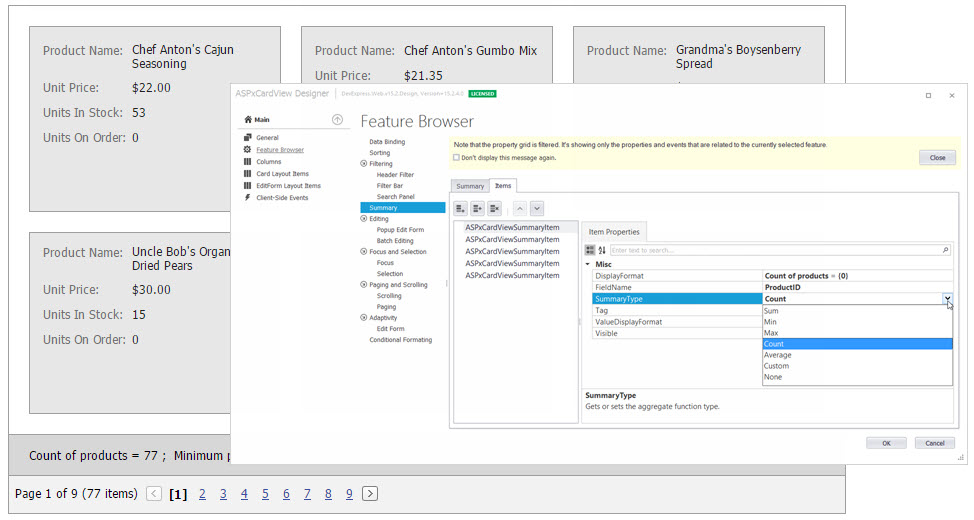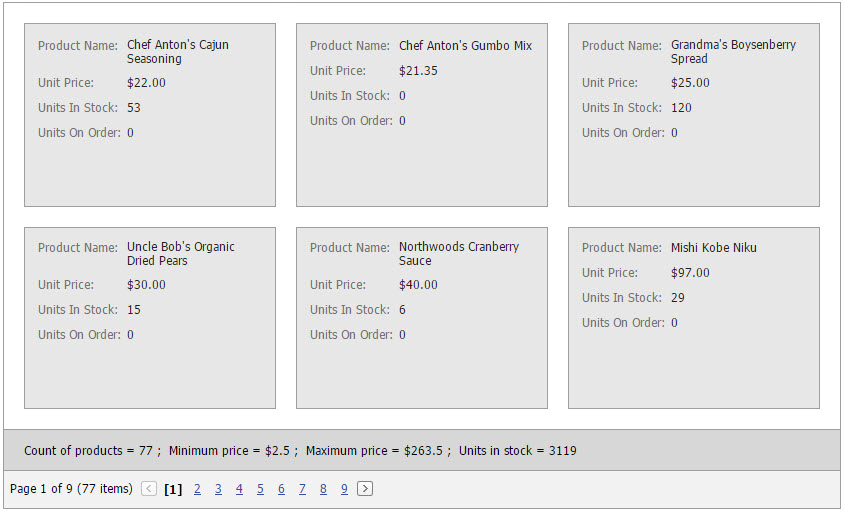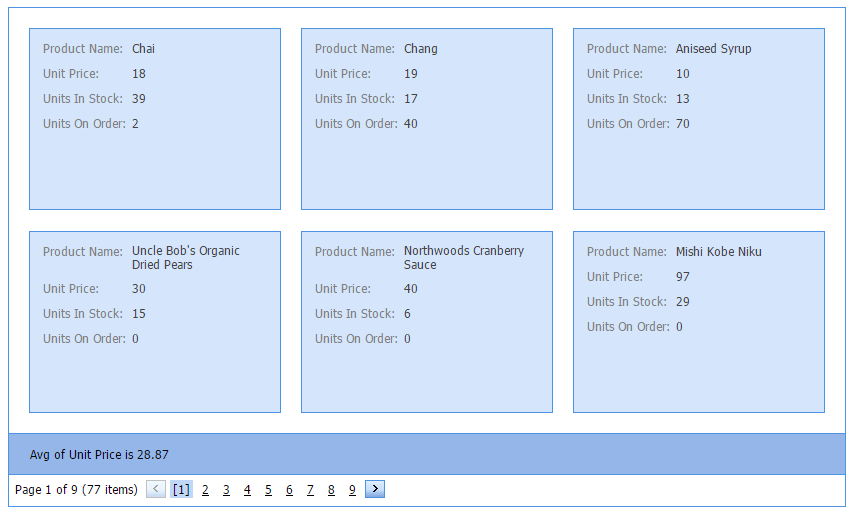20.2
20.1
19.2
19.1
18.2
18.1
17.2

# Total Summary

The total summary represents the value of an aggregate function calculated over all cards displayed within the ASPxCardView. Total summaries are displayed in the summary panel, provided that the ASPxCardViewSettings.ShowSummaryPanel option is enabled.Total summaries are represented by the ASPxCardViewSummaryItem objects. ASPxCardView stores its total summaries within the ASPxCardView.TotalSummary collection. To manage this collection at design time, you can use the Edit Form as shown above.

To obtain a total summary value, use the ASPxCardView.GetTotalSummaryValue method.

## Example: Creating Total Summaries in Markup

The code sample below demonstrates how you can specify total summaries.

The image below shows the result.``````<dx:ASPxCardView ID="ASPxCardView1"  runat="server" AutoGenerateColumns="False" DataSourceID="SqlDataSource1" KeyFieldName="ProductID">
<Settings ShowSummaryPanel="True" />
<Columns>
...
</Columns>
<TotalSummary>
<dx:ASPxCardViewSummaryItem FieldName="ProductID" SummaryType="Count" DisplayFormat="Count of products = {0} " />
<dx:ASPxCardViewSummaryItem FieldName="UnitPrice" SummaryType="Min" DisplayFormat="Minimum price = \${0} " />
<dx:ASPxCardViewSummaryItem FieldName="UnitPrice" SummaryType="Max" DisplayFormat="Maximum price = \${0} " />
<dx:ASPxCardViewSummaryItem FieldName="UnitsInStock" SummaryType="Sum" DisplayFormat="Units in stock = {0} " />
</TotalSummary>
</dx:ASPxCardView>
``````

## Example: Creating Total Summaries in Code

This example shows how to calculate the average price and display it in the ASPxCardView's summary panel:``````ASPxCardViewSummaryItem totalSummary = new ASPxCardViewSummaryItem();
totalSummary.FieldName = "UnitPrice";
totalSummary.SummaryType = DevExpress.Data.SummaryItemType.Average;